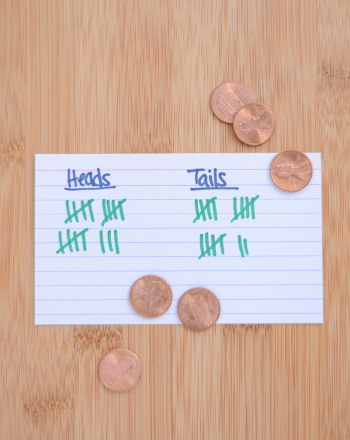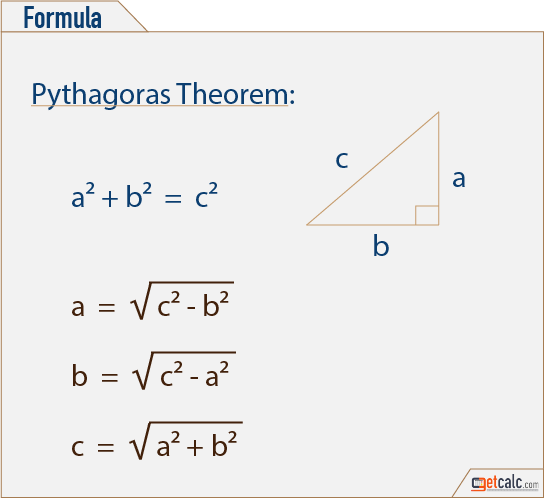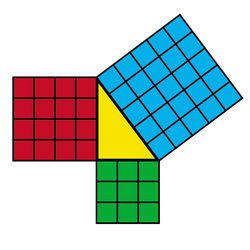Maths Project

Maths Projects - List of Math Project Models and Examples

Mathematics is a word that fears most of the students in their early grade until then and now.

The early graders normally fear the word "Mathematics". But in reality, math's is a subject full of logic and proofs.

This no longer is primary to teach the students in the 21st century. Including Mathematics Project in the upper primary stage aids students in picturing the fundamental concepts, principles, theorems, and the underlying procedure occupied in solving them. Mathematics is a word that is feared by the largest of the students in their early grade.
Following are some of the advantages of Math’s projects in our schools:

While traditional learning is not much suggested to solve the math problems, it is recommended to the teachers and board to grant math’s education in a more rational and challenging through project works.

It has been seen that rote learning is not effective for the long-term. Math’s related project work not only to help in improving the problem-solving ability but also will be able to learn it in a better way for their lifetime.

These projects help the students to improve the planning and critical thinking ability of the student as they employ “habit of thinking and mind skills”.

Including this concept in the curriculum will also help improve reasoning skills of the student.

Remember when you try to learn something relating to the real world you understand it better. Similarly, when you try to learn a concept with more examples and relating it to the real world the concept gets deeper into your mind and retains forever and ever. Henceforth, it is always good to do a project on the concept you have understood.

Project 1Probability:

Probability is such a native part of your life that you rarely think about it. Though, every time you use a word like “might,” “may,” “undoubtedly,” “without fail,” or “maybe,” you can see and even a probability that an event will occur.

Scientists and great mathematicians like to express probability more accurately. For example, if you toss a coin in the air, the probability (P) that it will land heads or tails.

Materials required:
A book and a pencil
Four coins

Procedure:

Using a paper and pencil, draw circles with an “H” or a “T” in the center of the paper to illustrate the different results when you toss these three coins.

Using the circles that you drew as mentioned above, express the following:

• • The probability of getting three heads while tossing the coins

• • The probability of getting three tails while tossing the coins

• • The probability of getting one head and three tails while tossing the coins.

• • The probability of getting one tail and three heads while tossing the coins.

• Hint: There are eight distinctly different possibilities so make sure you haven’t left any of them out.

Try tossing three coins 16 times and writing down the outcomes. Are the probabilities roughly equal as you calculated in step 2? Try tossing three coins 24 times. Are the probabilities any closer?

Project 2

Pythagoras Theorem:

This theorem states that the square on the hypotenuse of a right-angled triangle is equal in area to the sum of the squares on the other two sides.

Materials Required:
Color papers
Ruler and sketches
Procedure:
Make a right angle Triangle, of 3cm, 4cm and 5cm as shown 3cm 5cm
Make 3 square sheets of 3 * 3 cm, 4 * 4 cm, 5 * 5 cm.
Fix these square sheets to the sides of the triangle.
Make the square sheets into 3 * 3 cm such that 9 squares of equal length
Similarly, repeat the same thing with the remaining square sets. 4cm
By this work, we can prove the above-said theorem.In the above work, we have considered a= 3cm, b=4cm and c=5cm.
Henceforth, 3 square + 4 square = 5 square.
• • 3 * 3 + 4 * 4 = 5 * 5

• • 9 + 16 = 25

• • 25 = 25

• Hence the theorem is proved.

Assessment:

• 1. Geometry Map Project:

• Angles, Lines, and Triangles: Your assignment is to design a map that includes several distinct kinds of lines, angles, and triangles. Your map can be of a town, your neighborhood or a made-up place of your own. It must, however, incorporate the following: 2 sets of streets that are parallel Two sets of streets that are perpendicular One street that intersects another street to form an obtuse angle.

• Create a menu for a themed restaurant. The student will write word problems using their menu. It can be a very fundamental element, just adding prices of different items, to more complex problems, like finding the cost of a meal for you and a friend plus tax or figuring the cost of the meal for grandma with her senior discount of 10%. The criteria for each of the following items in the menu are as follows: 4 different types of appetizers 4 different types of beverages and 4 main dishes.

• 3. COMPARE TWO FRACTIONS:

• Two proper fractions are given to you with different denominators (show how to write these fractions as decimal numbers and as a percentage. Compare both the fractions using an appropriate mathematical symbol.) Be sure to explain what you are thinking!

• 4. SUPPLEMENTARY AND COMPLEMENTARY ANGLES:

• Label and highlight the given angles from 6 different pictures. Select six of the following angles: acute angle, obtuse angle, straight, right angle, supplementary angles, and complementary angle. Then measure the acute angle, obtuse angle, supplementary angle, and complementary angles, and write their measures inside the interior of each angle.

• 5. DESIGN YOUR DREAM HOUSE:

• Draw a 2D version of how you want the front of your house will look like. Must include four windows, and two doors. All lines must be drawn with the help of a ruler and must be in centimeters. Students must then determine the perimeter and area of each the window and the door. You must also do the same for the front of the house such that the perimeter and the area. Then you must convert these measurements to millimeters. Each dimension must be written in typical drafting fashion. Houses must be uniformly colored, and students may add additional features.

• 6. MATHEMATICS ARTICLE:

• Draft an article about mathematics, which may include a mathematician. Write 2
important things you learned from the article. At least one-page minimum. Note, write the